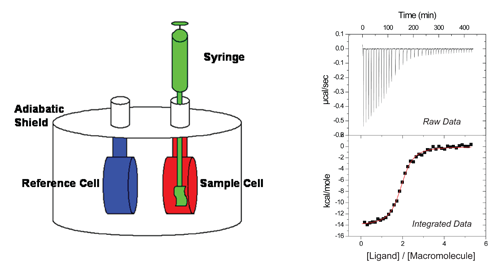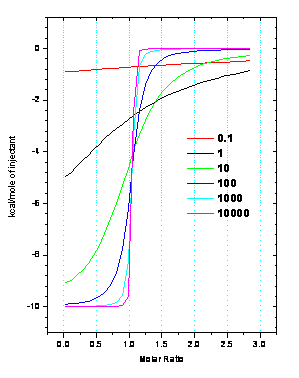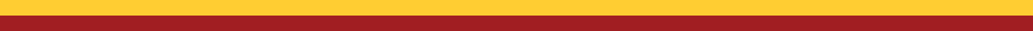﻿ Isothermal Titration Calorimetry

# Isothermal Titration Calorimetry

A comprehensive thermodynamic characterization of any single reaction is necessary to fully appreciate its impact on a system involving coupled reactions. Isothermal titration calorimetry (ITC) is now commonly used to study such reactions.  This is due in part to significant advances in technology over the last few decades enabling the development of very accurate calorimeters.  The increasing popularity of this technique is apparent when considering the results of a literature search for the keyword ‘ITC’, which shows a monotonic increase in publications over the last two decades.

Current models of ITC instruments consist of two identical coin-shaped Hastelloy® (or gold) cells, one being the sample cell and the other the reference cell, connected via a thermocouple and surrounded by an adiabatic jacket.  A heater is linked to each cell and a set amount of power is delivered to each to maintain thermal equilibrium. The macromolecule of interest is loaded into the sample cell while a solution of equal heat capacity, commonly an identical buffer lacking the macromolecule, is loaded into the reference cell.  The ligand of interest is then loaded in the titration pipet and inserted into the sample cell.  Very small volumes of titrant, typically 2-10 µL, are delivered to the sample cell.  As the reaction progresses, any difference in temperature between the sample and reference cells is detected by the thermocouple and the power delivered to the sample cell is adjusted accordingly (raw data in figure).  The injection enthalpy is determined directly from the raw data by integration of the power delivered to the cell with respect to time followed by concentration normalization.In a standard ITC experiment, a known concentration of ligand is injected into the reaction cell containing a known concentration of macromolecule.  The ensuing thermodynamic profile, describing the heat flow over the course of the experiment, is fit to a mathematical model using non-linear least-squares analysis.  Currently, there are several fitting models provided with the ITC software.

·         One-site.  This is the simplest binding model.  It assumes that n ligands bind per macromolecule with identical thermodynamics and the best-fit values of n, the reaction stoichiometry, K and ΔH are determined.

·
Two-site.  This model makes the assumption that there are two binding events that occur with independent thermodynamics and the statistical saturation of each site is dictated by the magnitude of the individual K values.  In this case, the best fit will generate n, K and ΔH for each site.  It is a common mistake to assume that n correlates with the x-axis position of the inflection, but each n value is independent of the other for this model (i.e. n1 = 1 and n2 = 2 means one ligand binds to site 1 while site 2 binds two ligands with equivalent thermodynamics).

·         Sequential.  This model requires that the 1st ligand to bind to the macromolecule goes to the 1st site, and the nth ligand to bind goes to the nth site.  The sequential saturation requisite excludes n as a fitting parameter since a non-integer value would make no physical sense.  For this reason, the accuracy of molecular concentrations is more important for the sequential model, as n is a soft parameter in the previous two models and can often correct for inaccurate concentrations.  A benefit of this model is the smaller number of fitting parameters, which makes it possible to obtain a unique fit for very convoluted data, assuming that each site has significantly different K and/or ΔH values.  It is essential to keep in mind that this model is not appropriate for independent sites where saturation is dictated by individual binding constants.

·
Competition. Utilization of this model is appropriate for situations that require the use of a competing ligand, for example when solubility is an issue or KITC is too large to accurately fit in a direct titration.  In contrast to the competition experiments to be discussed in detail later, this model assumes that the competing ligand is present only in the syringe, and its apparent concentration is calculated throughout the titration.

Anytime experimental data is fit to a mathematical model, there is an optimal range of values that maximize confidence in the fit parameters.  For ITC, this range is known as the c-window, 1 < c < 1000 , where c is defined numerically by

c = nKITC•[Macromolecule]As depicted in the figure, when c grows too large, the resulting ITC data takes on the shape of a step function and can be fit to any KITC ~ 109, although accurate ΔH data can be obtained. When c is too small, the data represents a featureless curve and can be fit to multiple n, K and ΔH values.

In many cases, ITC experiments are straightforward to design and data analysis is possible using the fitting models above with no further complications.  This is generally not the case when one of the species of interest is a metal ion.  In these situations, significant speciation is likely, resulting in additional experimental considerations and necessitating a more rigorous post-fit analysis to accurately account for all coupled equilibria.  This is of particular interest for our lab and is detailed here (or more consicely here).